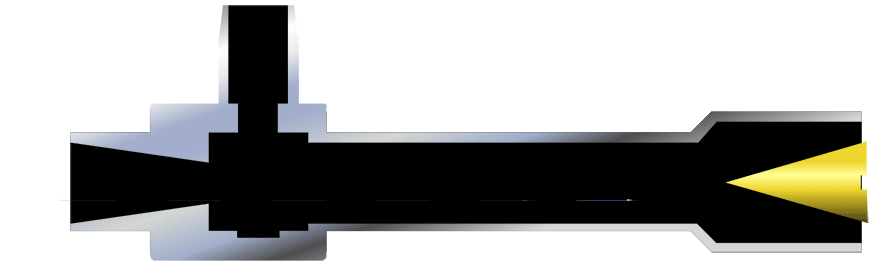## Vortex Tubes: What is a Cold Fraction & How to use it to Your Benefit

EXAIR has wrote many different articles about how Vortex Tubes work and the applications in which they are used.  The idea of making cold air without any freon or moving parts is a phenomenon.  This phenomenon can generate cold air to a temperature as low as -50 oF (-46 oC).  In this article, I will explain the adjustment of the Vortex Tube to get different temperatures and cooling effects in reference to the Cold Fraction.To give a basic background on the EXAIR Vortex Tubes, we manufacture three different sizes; small, medium, and large.  These sizes can produce a range of cooling capacities from 135 BTU/hr to 10,200 BTU/hr.  The unique design utilizes a generator inside each Vortex Tube.  The generator controls the amount of compressed air that can enter into the Vortex Tube.  As an example, a medium-sized Vortex Tube, model 3240, will only allow 40 SCFM (1,133 SLPM) of compressed air to travel into the Vortex Tube at 100 PSIG (6.9 bar).  While a small-sized Vortex Tube, model 3208, will only allow 8 SCFM (227 SLPM) of compressed air at 100 PSIG (6.9 bar).  EXAIR manufactures the most comprehensive range from 2 SCFM (57 SLPM) to 150 SCFM (4,248 SLPM).

After the compressed air goes through the generator, the pressure will drop to slightly above atmospheric pressure.  (This is the “engine” of how the Vortex Tube works).  The air will travel toward one end of the tube where there is an air control valve, or Hot Air Exhaust Valve.  This valve can be adjusted to increase or decrease the amount of air that leaves the hot end.  The remaining portion of the air is redirected toward the opposite end of the Vortex Tube, called the cold end.  By conservation of mass, the hot and cold air flows will have to equal the inlet flow as shown in Equation 1:

Equation 1: Q = Qc + Qh

Q – Vortex Inlet Flow (SCFM/SLPM)

Qc – Cold Air Flow (SCFM/SLPM)

Qh – Hot Air Flow (SCFM/SLPM)

Cold Fraction is the percentage of air that flows out the cold end of a Vortex Tube.  As an example, if the control valve of the Vortex Tube is adjusted to allow only 20% of the air flow to escape from the hot end, then 80% of the air flow has to be redirected toward the cold end.  EXAIR uses this ratio as the Cold Fraction; reference Equation 2:

Equation 2: CF = Qc/Q * 100

CF = Cold Fraction (%)

Qc – Cold Air Flow (SCFM/SLPM)

Q – Vortex Flow (SCFM/SLPM)

EXAIR created a chart to show the temperature drop and rise, relative to the incoming compressed air temperature.  Across the top of the chart, we have the Cold Fraction and along the side, we have the inlet air pressure.  As you can see, the temperature changes as the Cold Fraction and inlet air pressure changes.  As the percentage of the Cold Fraction becomes smaller, the cold air flow becomes colder, but also the air flow becomes less.  You may notice that this chart is independent of the Vortex Tube size.  So, no matter the generator size of the Vortex Tube that is used, the temperature drop and rise will follow the chart above.

How do you use this chart?  As an example, a model 3240 Vortex Tube is selected.  It will use 40 SCFM of compressed air at 100 PSIG.  We can determine the temperature and amount of air that will flow from the cold end and the hot end.  The inlet pressure is selected at 100 PSIG, and the Hot Exhaust Valve is adjusted to allow for a 60% Cold Fraction.  Let’s use an inlet compressed air temperature to be 68 oF.  With Equation 2, we can rearrange the values to find Qc:

Qc = CF * Q

Qc = 0.60 * 40 SCFM = 24 SCFM of cold air flow

The temperature drop from the chart above is 86 oF.  If we have 68 oF at the inlet, then the temperature is (68 oF – 86 oF) = -18 oF.  So, from the cold end, we have 24 SCFM of air at a temperature of -18 oF.  For the hot end, we can calculate the flow and temperature as well.  From Equation 1,

Q = Qc + Qh or

Qh = Q – Qc

Qh = 40 SCFM – 24 SCFM = 16 SCFM

The temperature rise from the chart above is 119 oF.  So, with the inlet temperature at 68 oF, we get (119 oF + 68 oF) = 187 oF.  At the hot end, we have 16 SCFM of air at a temperature of 187 oF.

With the Cold Fraction and inlet air pressure, you can get specific temperatures for your application.  For cooling and heating capacities, these values can be used to calculate the correct Vortex Tube size.  If you need help in determining the proper Vortex Tube to best support your application, you can contact an Application Engineer at EXAIR.  We will be glad to help.

John Ball
Application Engineer
Email: johnball@exair.com